# The CrossTable Concept

In this tutorial we'll cover the idea behind the CrossTable. In the next article we'll walk you through creating a CrossTable from some sample data.

For a quick review of what a Pivot table is and how they work, head over to the Pivot tables review article.

In Chartnado, the CrossTable concept expands on the basic idea of the Pivot table. To recap, a pivot table provides the following things:

1. They are a way to easily group rows of data
2. Those groups can be created in combinations of Rows and/or Columns
3. For each intersection of Row and Column in the Pivot table, the source rows are filtered to only include the appropriate data
4. For the resulting rows one or more summary calculations are performed by dragging column names to Values
5. The output of the calculations are then visualized as cells in the Pivot table itself

The important point is #3: The source rows are filtered to only include the relevant values for Row and Column in the pivot structure, but the result of that filtering effectively gives you a new table.

With a CrossTable you can take the rows that are the result of the filtering from the intersection of each row and column in the pivot structure and make a complete visual, like a chart.

So to go back to our pivot table example, suppose we had the following data in an Excel data table: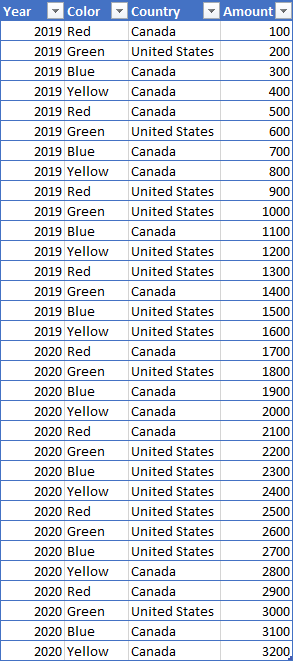From the Excel pivot table article we created a basic pivot table by dragging Year to Rows, Color to Columns and Amount to Values, giving us the following, basic pivot table: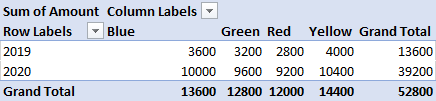Now from our discussion of pivot tables we know that the number 3200 at the intersection of Year: 2019 and Color: Green comes from the fact that the original data was effectively filtered to only include those rows before the calculation for sum of Amount was done, giving us these rows: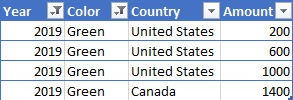However, those rows contain more information than can be further grouped and calculations made from it, if used appropriately. That fact is the basis for the CrossTable.

With Chartnado instead of just being able to visualize numbers in each pivot cell we can make a whole chart. So, if we were to again use the values [2019, Green] in our example you could make a bar chart that showed the total sales for Canada vs the United States, like so: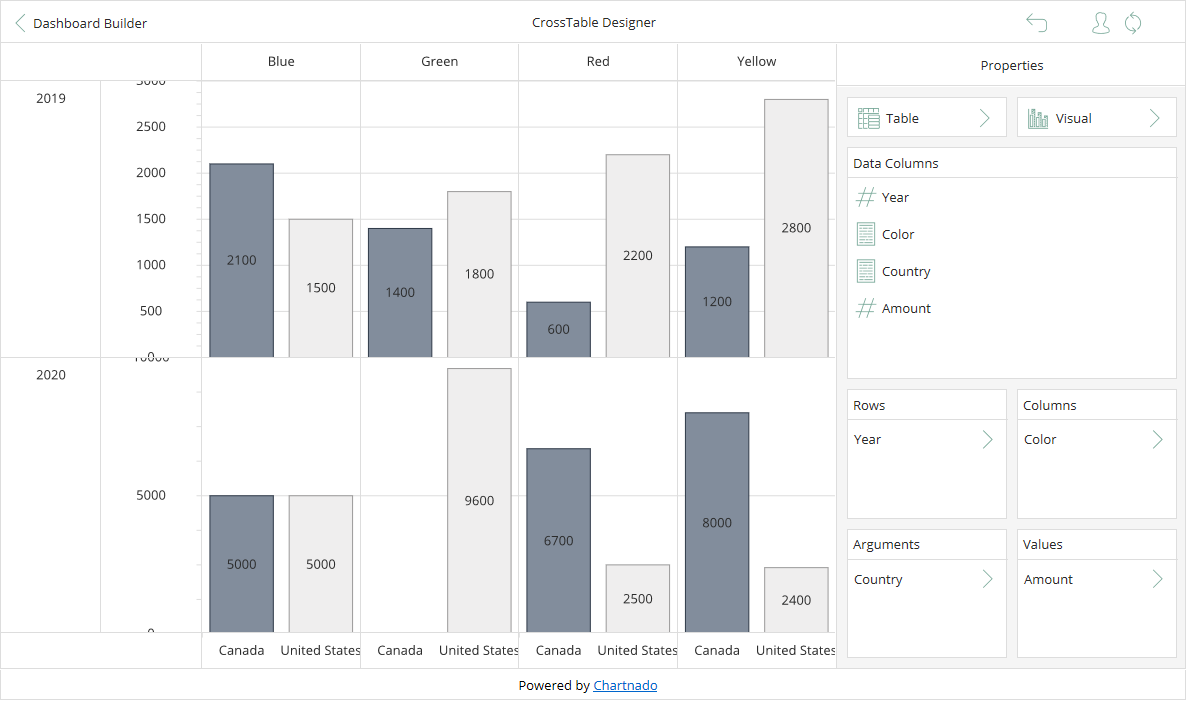In this CrossTable we have arranged the grouping of the data like we did in the pivot table, with Year in the Rows area, Color in the Columns area and Amount in the Values area. Note that just like for the pivot table there are two rows, one for each year and four columns, one for each color. The difference is now that for each cell in the CrossTable we have a chart.

If we examine the cell for our [2019, Green] example you can see the chart for that cell: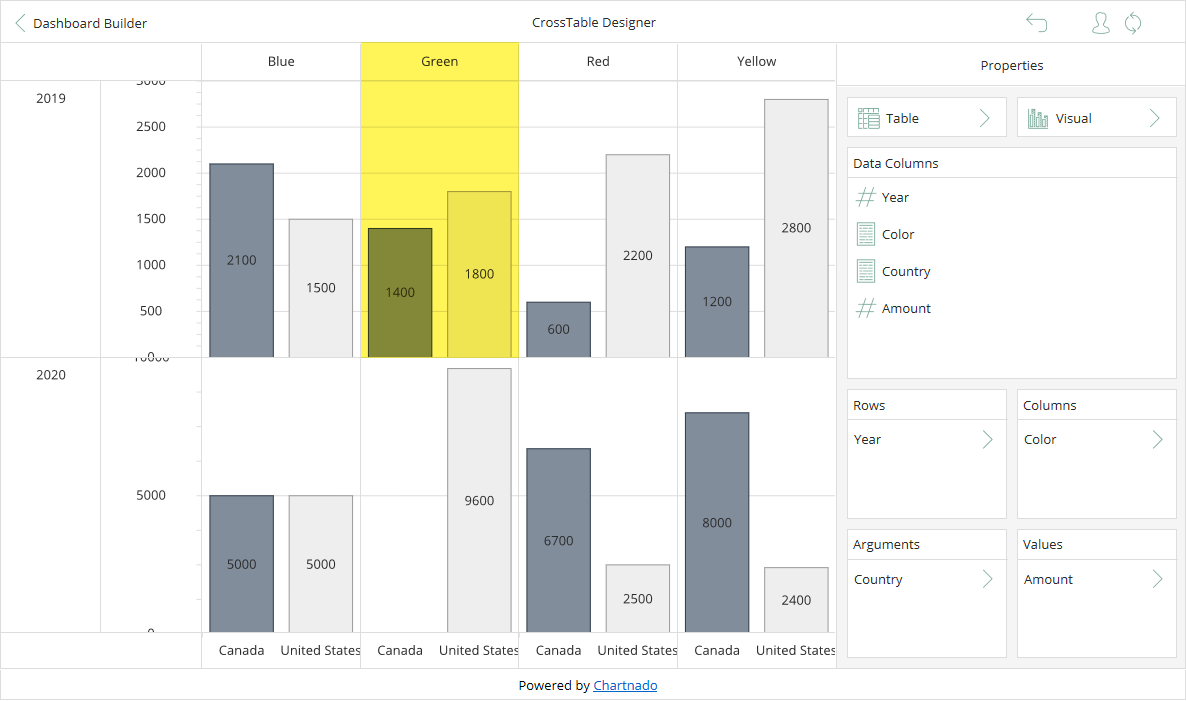And, if you go back to the data you'll notice that the sum of Amount for Canada is 1400 and the sum of Amount for United States is 1800.